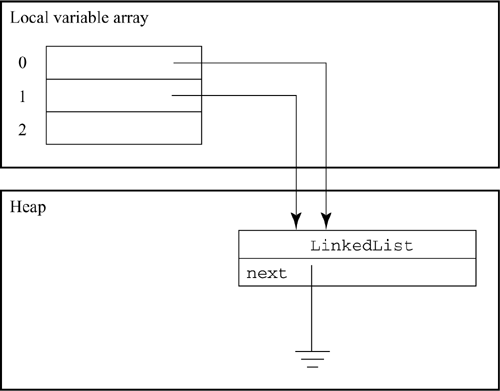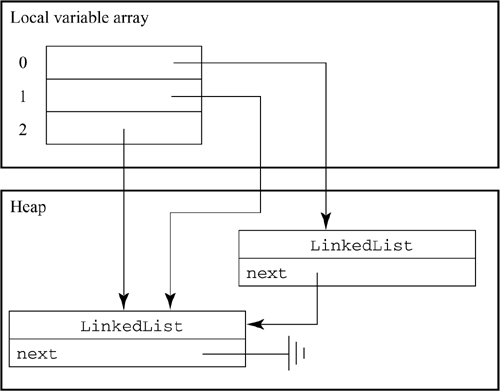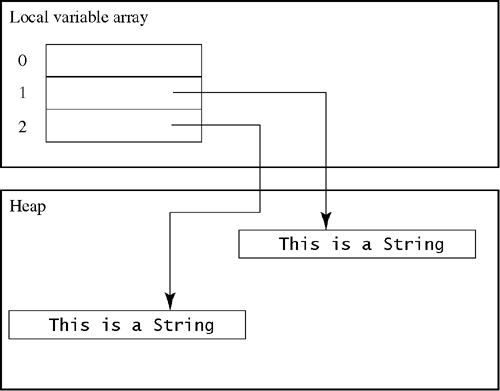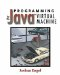# 10.11 Ifs and Booleans

Java's type boolean has two values: true and false. Though booleans may be stored in variables, they are primarily used as the conditions for if, while, and for statements.

#### 10.11.1 Boolean Values

Within JVM programs, the Java type boolean is represented by an int, with 1 representing true and 0 representing false. The expression true compiles to

` iconst_1 `

and false to

` iconst_0 `

Consider this statement:

` debug = false; `

The expression false is pushed onto the stack, then assigned to the boolean variable debug (in variable 1, for example):

` iconst_0              ; Push false istore_1              ; Store into debug `

You can test boolean values with ifne, which will branch on true values, or ifeq, which will branch on false values. Remember that ifne and ifeq compare to zero, so you can read ifeq as "Branch if the top of the stack is equal to false" and ifne as "Branch if the top of the stack is equal to true." This is a simple if statement:

` if(debug) {    // Print debugging information } `

This statement translates into

` iload_1               ; Get the value of debug ifeq end_of_if        ; Skip debugging if debug is false ;; The compound statement to print debugging information goes here end_of_if:            ; Continue here `

If debug is false, then the ifeq test succeeds, and control continues at end_of_if. If debug is true, then the test fails, and control continues with the next instruction. The next instruction is the compiled code to print debugging information. After printing debugging information, control proceeds to end_of_if.

If the if statement includes an else, then there are two different substatements. One should be executed if the test succeeds, the other if the test fails. Both statements must be compiled. For example,

` boolean weekend; if(weekend)    sleep_late(); else    go_to_school(); `

Suppose we assign variable 1 to hold weekend. This code compiles as

` iload_1                       ; Push the value of weekend ifne true_case                ; If it's not 0,                               ; then go to the true case false_case:                   ; Do this on failure    invokestatic Student/go_to_school()V    goto end_of_if             ; Skip to the end of the if true_case:                    ; Do this on success    invokestatic Student/sleep_late()V end_of_if:                    ; Continue here in either case `

There are two cases here, represented by the code at true_case and false_case. The program should execute one or the other depending on the value of the boolean expression weekend. If weekend is true, then control skips over the false_case and goes to true_case. If weekend is false, control continues at the false_case.

At the end of the false_case code, a goto causes the program to skip to end_of_if. The program will also end up at end_of_if if it went to true_case. If the goto weren't there, the program would try to both sleep_late and go_to_school, which any student will tell you is impossible.

#### 10.11.2 if Statements

The general form of an if statement is

` if(test-expression)    true-statement else    false-statement `

The general pattern for compiling an if statement is

` code to evaluate test-expression branch to true_case if expression is true    code to execute false-statement    goto end_of_if true_case:    code to evalute true-statement end_of_if: `

We have already discussed how to compile the expression and the substatements. There are many different ways the branch can be compiled, depending on the form of test-expression. The next sections discuss this in more detail.

#### 10.11.3 Comparisons

According to the Java language, expressions that involve comparison operators, such as <, >=, and ==, evaluate to boolean values:

` float temperature = check_thermometer(); boolean freezing = temperature < 32.0; `

This code assigns true to freezing if the temperature is below 32 degrees Fahrenheit, false otherwise. The code to compute the value of the expression temperature < 32.0 is

` .var 1 is temperature F fload_1              ; Push the temperature ldc 32.0             ; Push 32 degrees fcmpgl           ; Compare the two iflt true_case       ; Go to the true case iconst_0             ; Push false goto end_of_if       ; Skip over the true case true_case:           ; Go here if true iconst_1             ; Push true end_of_if:           ; The flow of control ends up here                      ; Either 1 or 0 is now atop the stack `

There are two paths through this code, depending on the value of temperature. Either way, the stack height will increase by 1 at the end of this code. The top of the stack will contain either true or false. Nothing else on the stack has changed. That's what you'd expect from code for an expression.

This may seem like a lot of jumping around for a relatively simple expression. Usually, boolean values are not represented explicitly. Instead, comparisons are usually done as part of an if, while, or for statement. Consider this code:

` if(temperature < 32.0)    predict_snow(); else    predict_rain(); `

It's never necessary to actually compute the boolean value of the comparison. Instead, the compiler uses the result of the fcmpg instruction directly to branch to either predict_rain or predict_snow:

` fload_1               ; Push the temperature ldc 32.0              ; Push 32 degrees fcmpgl                ; Compare the two iflt true_case        ; Go to the true case if temp < 32 invokestatic Weatherman/predict_rain()V      ; Call for rain goto end_of_if        ; Skip over the true case true_case: invokestatic Weatherman/predict_snow()V      ; Call for snow end_of_if:            ; Continue here `

The fcmpg instruction puts 1 on the stack if the temperature is less than 32, 0 if it's exactly equal to 32, or 1 if it's greater than 32. The test succeeds if the temperature is less than 32, which makes the result of fcmpg less than 0.

This technique works for double and long values as well, using dcmpl or lcmp, respectively. For example, with long values,

` // Get the current time as the number of milliseconds from // January 1, 1970. long now = System.currentTimeMillis(); if(now >= 978307200000)    System.out.println("Welcome to the 21st century"); `

This code compiles as

` invokestatic java/lang/System/currentTimeMillis()J ldc2_w 978307200000 lcmp                      ; Compare now to the first msec                           ; of the 21st century iflt end_of_if            ; If we're not there yet, skip                           ; to the end of the if getstatic java/lang/System/out Ljava/io/PrintStream; ldc "Welcome to the 21st century" invokevirtual println(Ljava/lang/String;)V end_of_if: `

The particular kind of branch instruction used depends on the comparison and whether or not there's an else case. The ifne mnemonic stands for "Skip if not equal," the opposite meaning of ==, and ifle stands for "Skip if less than or equal to," the opposite meaning of >. The if operator is chosen as the opposite of what the mnemonic suggests: ifne for ==, ifle for >, and so on. That's because the if is being used to skip over the code to be executed if the test succeeds. It's like a double negative making a positive: "Skip over this code if the opposite of the test is true" is the same as "Execute this code if the test is false."

#### 10.11.4 Floating-Point Comparisons and NaN

In addition to affecting the type of if chosen, the operator also affects the instruction used for the comparison. If the comparison is a < b or a <= b, where a and b are both doubles, the dcmpg instruction is used; otherwise, the dcmpl instruction is used. Generally, dcmpg is used in conjunction with ifgt or ifge, and dcmpl is used in conjunction with iflt or ifle.

In ordinary cases, dcmpl and dcmpg are identical. The difference is seen when either a or b turns out to be not-a-number (NaN). NaNs are the result of an undefined calculation, such as 0/0. All comparisons to NaN should fail; that is, this program

` double not_a_number = 0.0/0.0;      // Results in NaN if(not_a_number < 0)    System.out.println("0/0 < 0"); if(not_a_number > 0)    System.out.println("0/0 > 0"); if(not_a_number == 0)    System.out.println("0/0 == 0"); `

doesn't print anything, since NaN is not less than, greater than, or equal to 0. This is how the code compiles:

` dconst_0                   ; Compute 0/0 (NaN) dconst_0 ddiv dstore_1                   ; Store NaN in 1 and 2 dload_1                    ; Push the NaN dconst_0                   ; Compare it to 0 with dcmpg dcmpg                      ; This leaves 1 ifge end_of_if1            ; Skip, since 1 >= 0 ;; Print the message 0/0 < 0 end_of_if1: dload_1                    ; Push the NaN dconst_0                   ; Push 0 dcmpl                      ; This time, the result is -1 ifle end_of_if2            ; Skip again, since -1 <= 0 ;; Print the message 0/0 > 0 end_of_if2: dload_1                    ; Push the NaN dconst_0                   ; Push 0 dcmpl                      ; This time, the result is -1 ifne end_of_if3            ; Skip again, since -1 != 0 ;; Print the message 0/0 == 0 end_of_if3: `

In the first comparison, dcmpg leaves 1 on the stack if either operand is NaN. Since 1 >= 0, the ifge treats the comparison as success, so it skips over the code. This is another of those two-negatives-make-a-positive situations: the comparison succeeds, so it doesn't do the code inside the if statement.

Similarly, the second comparison uses dcmpl. Since dcmpl leaves 1 on the stack, the comparison succeeds, so the print code is not executed.

In the third case, where the comparison is ==, either dcmpl or dcmpg could be used; it is up to the discretion of the compiler writer. The results are the same no matter which instruction is chosen.

There is a similar distinction between fcmpl and fcmpg. There's only one form of lcmp, since there's no way for a long to be not-a-number. When a long value is divided by 0, an ArithmeticException is thrown instead of resulting in NaN.

#### 10.11.5 Integer Comparisons

Compiling int and reference comparisons is different from compiling double, float, and long comparisons. In the interests of efficiency, the compare instruction and the branch instruction have been rolled into one. For example, suppose that i is an int in

` if(i < 10) {    // Do something } `

This code compiles to

` iload_1            ; Push i from variable 1 bipush 10          ; Push the constant 10 if_icmpge done     ; If i >= 10, skip the next code ;; Do something done:              ; Control continues here `

The < operator translates into a single if_icmpge instruction, which does both the comparison and the branch.

#### 10.11.6 References and Object Equality

The Java == operator, when applied to two expressions that evaluate to objects, is true when the two references point to exactly the same object or if both are null, false otherwise. For example,

` LinkedList head = new LinkedList(); LinkedList tail = head; `

This code compiles to

` new LinkedList        ; Create a new linked list dup                   ; Initialize it invokespecial LinkedList/<init>()V astore_0              ; Store it in head (var 0) aload_0               ; Retrieve the value                       ; Now the same reference is found both                       ; in var 1 and on the stack astore_1              ; Now both var 0 and var 1 refer to                       ; the same object `

Variables 0 and 1 (head and tail, respectively) point to the same object; the references they contain are identical. The JVM variable pool and heap look like the diagram in Figure 10.4. Currently, head == tail is true, since they both point to the same object. However,

` head.next == tail `
##### Figure 10.4. After creating head and tailis false, because the left side is null and the right side is non-null. References to null are equal only to themselves, which means that

` head.next == tail.next `

is true, not because head == tail but because both head.next and tail.next are both null.

Suppose you add a new element to the end linked list:

` Object new_item = new LinkedList(); tail.next = new_item; tail = new_item; `

This code compiles as

` aload_1                    ; Push tail new LinkedList             ; Create the new link dup invokespecial LinkedList/<init>()V astore_2                               ; Put it in variable 3                                        ; temporarily aload_2                                ; Get it back astore LinkedList/next LLinkedList;    ; Store the new link in                                        ; the next of the current                                        ; tail aload_2 astore_1                               ; Set tail to the new link `

This code changes what tail in variable 1 points to. The JVM state is diagrammed in Figure 10.5. Now head and tail point to different objects. The next field of the original element is now set to the new element, instead of to null. For that reason,

` head == tail `
##### Figure 10.5. After adding a new elementwill result in false, because they point to different objects. However,

` head.next == tail `

will result in true, because both point to the same object (the new one).

Note that two objects created with new are always different objects, even if the values of the objects are the same. The code fragment

` String s1 = new String("This is a String"); String s2 = new String("This is a String"); if(s1 == s2)    System.out.println("The two Strings are identical"); else    System.out.println("The two Strings are NOT identical"); `

will always print

` The two Strings are NOT identical `

This code compiles to

` new java/lang/String           ; Build the first string dup ldc "This is a String" invokespecial java/lang/String/<init> (Ljava/lang/String;)V astore_1                       ; Store it in variable 1 new java/lang/String           ; Build the second string dup ldc "This is a String" invokespecial java/lang/String/<init> (Ljava/lang/String;)V astore_2                       ; Store it in variable 2 aload_1                        ; Compare them aload_2 if_acmpne false_case: getstatic java/lang/System/out Ljava/io/PrintStream; ldc "The two Strings are identical" invokevirtual java/io/PrintStream/println (Ljava/lang/String;)V goto cont: false_case: getstatic java/lang/System/out Ljava/io/PrintStream; ldc "The two Strings are NOT identical" invokevirtual java/io/PrintStream/println (Ljava/lang/String;)V cont: `

After executing this code, memory looks like the diagram in Figure 10.6. The references can be compared only to see whether or not they are equal. Use if_acmpeq and if_acmpne with references exactly as you'd use if_icmpeq and if_icmpne on ints. It is meaningless to say that one reference is "less than" or "greater than" another. The Java language permits you to use == and != on references but not <, >, <=, or >=. The instructions if_acmplt, if_acmpgt, if_acmple, and if_acmpge do not exist.

##### Figure 10.6. Two different StringsIf you want to compare two objects to see if their contents are equal, instead of being identical references, use the method equals. The implementor of the class provides this method, because only the person who wrote the class knows exactly what is meant by the two objects having equal contents.

The definition of equals in Object returns true only if the two references are identical. This method definition is inherited by all other classes unless it is overridden by the implementor. For example, the class String has a definition of equals that is true if the strings have identical sets of characters.

You can compare the two strings in the example code to see whether they have identical text with

` aload_1               ; Push the first string aload_2               ; Push the second string invokevirtual java/lang/Object/equals(Ljava/lang/Object;)Z `

This code will leave 1 on the stack if they are equal, 0 if they are not. In this case they are equal, since they have the same text, even though variable 1 and variable 2 point to different objects. You must be careful here: if variable 1 contains null, this code will throw a NullPointerException. You must check for this case separately.

#### 10.11.7 Boolean Operators

Java's && and || operators work much like ifs: the second operand is not evaluated if the first argument makes it unnecessary. Consider

` class Demo {    static boolean a()    {        System.out.println("In a");        return false;    }    static boolean b()    {        System.out.println("In b");        return true;    }    public static void main(String args[])    {        System.out.println("a && b == " + (a() && b()));    } } `

When this class is executed (that is, main is run), it prints

` In a a && b == false `

b() is never called; if it had been, the message "In b" would have been printed. That's because a() returned false. It doesn't matter what b returns, since false && anything is false. This is called shortcutting. Shortcutting is required by the Java language; if the compiler had generated code that printed both In a and In b, the compiler would have been wrong.

The expression

` a() && b() `

compiles into JVM code as

` invokestatic Demo/a ()Z   ; Evaluate a() ifeq false_case           ; Shortcut over b() if a() fails invokestatic Demo/b ()Z   ; Evaluate b() ifeq false_case           ; Go to true_case only if both true_case:                ; a() and b() return true    iconst_1               ; Push 1 if true    goto end false_case:    iconst_0               ; Push 0 if false end:                      ; The rest of the program `

A similar form of shortcutting occurs with the || operator, except that the shortcut occurs if the first operand is true. The expression

` a() || b() `

compiles to

` invokestatic boolean_demo/a ()Z         ; Evaluate a() ifne true_case            ; Shortcut over b() if a() succeeds invokestatic boolean_demo/b ()Z         ; Evaluate b() ifne true_case            ; Go to false_case only if both false_case:               ; return false    iconst_0               ; Push 0 if false    goto end               ; Skip the true case true_case:    iconst_1               ; Push 1 if true end:                      ; The rest of the program `

If || and && expressions are used inside an if statement, then the iconst_0 and iconst_1 instructions used above are replaced by the success and failure statements of the if statement.Programming for the Javaв„ў Virtual Machine
ISBN: 0201309726
EAN: 2147483647
Year: 1998
Pages: 158
Authors: Joshua Engel

Similar book on Amazon

flylib.com © 2008-2017.
If you may any questions please contact us: flylib@qtcs.net